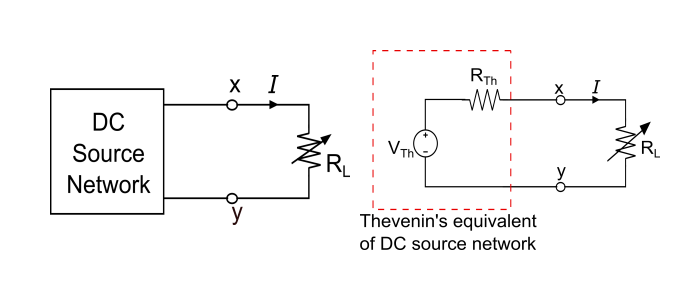# Maximum Power Transfer Theorem

Electronics & ElectricalDigital ElectronicsMiscellaneous

The maximum power theorem (MPT) is used to find the value of load resistance for which there would be maximum amount of power transfer from the source to load.

## Statement of MPT

A resistive load that is connected to a DC source, receives maximum power when the load resistance is equal to the internal resistance of the source as seen from the load terminals.

## Explanation of MPT

Consider the following circuit diagram to determine the value of RL such that it receives maximum power from the DC source.$$I=\frac{V_{Th}}{R_{Th}+R_{L}}$$

Thus, the power delivered to the resistive load is,

$$P_{L}=I^{2}R_{L}=(\frac{V_{Th}}{R_{Th}+R_{L}})^{2}R_{L}$$

As we know, the load resistance is variable. Thus, PL can be maximised by varying RL.

$$\Rightarrow\:P_{L}=\frac{V_{Th}^{2}R_{L}}{(R_{th}+R_{L})^{2}}=\frac{V_{Th}^{2}R_{L}}{R_{Th}^{2}+R_{L}^{2}+2R_{Th}R_{L}}$$

$$\Rightarrow\:P_{L}=\frac{V_{Th}^{2}}{(\frac{R_{th}^{2}}{R_{L}}+R_{L}+2R_{Th})}=\frac{V_{Th}^{2}}{D}$$

In order to PL be maximum the denominator (D) term should be minimum, i.e.,

$$\frac{dD}{dR_{L}}=0$$

$$\frac{d}{dR_{L}}(\frac{R_{Th}^{2}}{R_{L}}+R_{L}+2R_{Th})=0$$

By solving the above differential equation, we get,

$$R_{L}=R_{Th}$$

Hence, maximum power will deliver to the load if the load resistance is equal to internal resistance of the source network i.e.

Load Resistance = Internal Resistance of the source

## Amount of Maximum Power (Pmax)

$$P_{max}=\frac{V_{Th}^{2}}{4R_{Th}}$$

Here, Pmax is the amount of maximum power consumed by the load.

The total power supplied by the source is

$$P=2\frac{V_{Th}^{2}}{4R_{Th}}=\frac{V_{Th}^{2}}{2R_{Th}}$$

The efficiency of the circuit during maximum power transfer becomes,

$$\eta=\frac{P_{max}}{P}\times\:100=50$$%

## Steps for Solution of Network using MPT

step 1 – Remove the load resistance and replace all the independent sources by their internal resistance and determine the RTh of the source network looking through the open circuited load terminals.

Step 2S – As per MPT, the value of RTh gives the value of load resistance RL i.e. RTh = RL, that allows maximum power transfer.

Step 3 – Find the value of VTh across the open circuited load terminals.

Step 4 – The amount maximum power transferred is given by,

$$P_{max}=\frac{V_{Th}^{2}}{4R_{Th}}$$

## Numerical Example

Find the value of load resistance RL in the circuit given below such that maximum power transfer takes place. Also, calculate the amount of maximum power.Solution

Step 1 – Remove the load resistance and replace all the independent sources by their internal resistance (in this case, 12 V ideal voltage source is short circuited) and determine the value of RTh that give RL corresponding to the maximum power transfer.$$R_{Th}=1\:\Omega\:\lVert\:6\:\Omega=\frac{1\times\:6}{1+\:6}=\frac{6}{7}\:\Omega$$

As per maximum power transfer theorem,

$$R_{L}=R_{Th}=\frac{6}{7}\:\Omega$$

Step 2 – Determine the VTh across the open circuited load terminals,Here, the current in the circuit is,

$$I=\frac{12}{1+6}=\frac{12}{7}\:Ampere$$

As, the load terminal is open circuited, hence the VTh is the voltage drop across the 6 Ω resistor. Thus,

$$V_{Th}=I\times\:6\Omega=\frac{12}{7}\times\:6=\frac{72}{7}\:Volt$$

The amount of maximum power delivered to the load is,

$$P_{max}=\frac{V_{Th}^{2}}{4R_{Th}}=\frac{(72/7)^{2}}{4\times\:(6/7)}=30.857W$$

Hence, the value of load resistance for maximum power transfer is equal to (6/7 Ω ) and the amount of maximum power delivered to the load is 30.857 Watt.